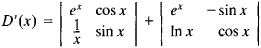# Functional Determinant

Also found in: Wikipedia.

## Functional Determinant

a determinant whose elements are functions of one or more variables.

The most important examples of functional determinants are the Wronskian, which plays an important role in the theory of linear differential equations of higher order, the Hessian, which is used in the theory of algebraic curves, and the Jacobian, which is used in transforming multiple integrals, in establishing the independence of a system of functions, and in other problems of the theory of functions of several variables.

The derivative of a functional determinant D(x) = |aik(x)| of order n is the sum of the h functional determinants whose matrices are obtained from the matrix ‖aik(x)‖ by differentiation of the elements of the first, second, . . ., nth column. For example, ifthenThe term “functional determinant” is sometimes used to refer to the Jacobian.

References in periodicals archive ?
In terms of elementary linear algebra, the existence of the coefficients [a.sub.l,m] and [b.sub.l,m] is equivalent to finding the zeros of the following functional determinant:
In general, the solution [y.sub.l](r; k) depends on the scattered direction x whenever entering the perturbation, so we denote the extended solution of (13) as [[??].sub.l](r; k) and accordingly the functional determinant as [[??].sub.l](k; [r.sub.1]).
More importantly, the functional determinant [MATHEMATICAL EXPRESSION NOT REPRODUCIBLE IN ASCII] is an algebraic condition that filters out a discrete set of eigenvalues from C for the problem
The functional determinant [[??].sub.l](k; r) is of order one and of type [MATHEMATICAL EXPRESSION NOT REPRODUCIBLE IN ASCII].
To study the functional determinants [[??].sub.l](k; r), we collect the following asymptotic behaviors of [[??].sub.l](r; k) and [[??]'.sub.l][(r; k).
Multiplying the argument of the [delta]-function by N leads to a functional determinant that is discussed in detail in the next paragraph:
Functional Determinant. A straightforward way of writing the functional determinant is by using anticommuting ghost fields
If the functional determinant is expressed in terms of ghost fields, this yields an extra symmetry, also called BRS-invariance .
The functional determinant of these transformations is field independent and can thus be omitted.
This equation is general enough to cover our intended applications, including different ways of considering the functional determinant (33).
Developing approaches to rational design of potential peptide inhibitors of structural functional determinants of proteins can potentially expand the range of therapeutic medications based on peptides .

Site: Follow: Share:
Open / Close